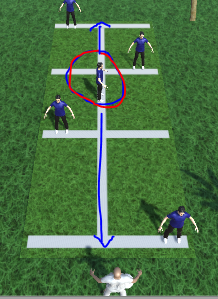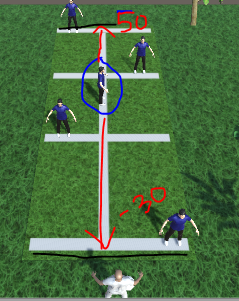# How to add end of path in AIpath

How to add start point and end of path to npc in Ai path?

I use Aipath to make the npc follow the target
but i want npc just move up and down without out of bounds
so i want to add start and end point to AIHi

You will have to create some script that changes the target for the AI.
Something like (pseudocode)

`````` if (target == top target && distance to target < 1) {
target = bottom target
}
if (target == bottom target && distance to target < 1) {
target = top target
}``````

thanks for ur answer, but can you tell me where i have to add that script in AI path ?
i want to add coordinate between 50 to -30 (axis z) as boundary point in my enemypls, help me…

Hi

A script that looks something like this should work. It should be attached to the same object as the AIPath script.

``````public class Patrol : MonoBehaviour {
public Transform[] targets;
int index;
AIPath agent;

void Awake () {
agent = GetComponent<AIPath>();
}

// Update is called once per frame
void Update () {
if (targets.Length == 0) return;
agent.target = targets[index];

if (targets.Length > 0 && Vector3.Distance(agent.target.position, transform.position) < 1)) {
index = (index + 1) % targets.Length;
}
}
}``````

but ,where i have to input coordinate in patrol script ?

i want my npc follow a target only in axis z 50 to -30 as bound point.

Hi

I suggest you take a look at some tutorials for how to use Unity before you try to use a large package such as this one.
See https://unity3d.com/learn/tutorials